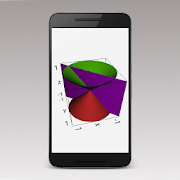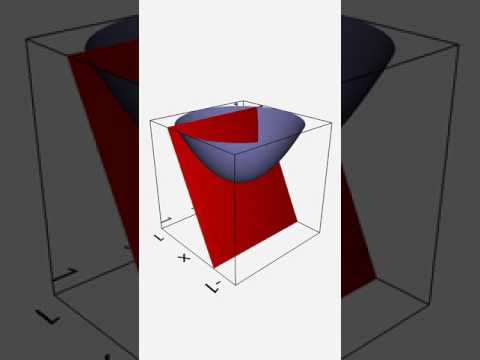# Graphing CalculatorEveryone
709
Contains AdsGraphing calculator, free and with no ads displayed on the main graph screens.
Super fast algorithms allow scrolling and zooming of 2D graphs in real time, using the touch sensitive screen.
3D graphs can be calculated quickly and then viewed from any angle.
Graph implicit equations in both two and three dimensions. e.g. x²+y²=5² or 5x+2y-3z=15.
Graph implicitly defined inequalities in two dimensions. e.g. 2x+5y<20.
Display up to 5 graphs on the same screen.
Active analysis of functions, for better graphing of 2D functions with singularity points. e.g. y=tan(x) or y=1/x.
Full scientific calculator.
Calculate complex numbers and matrices.
Comprehensive statistics functions.
Customization of all calculator and graph colors.

A fully ad free version of this app is also available.

The Graphing Calculator's functions include:

• numerical integration and differentiation of functions, with the results shown graphically.

• find roots, points of intersection and turning points of functions on the graph.

• key points, limits of integration, etc. can be selected by tapping on the graph or using a key pad to enter exact positions.

• pinch zoom and scroll all 2D graphs using the touch sensitive screen.

• draw tables of functions.

• rotate 3D graphs using the touch sensitive screen.

• statistics mode, including a full statistics summary with confidence intervals and Chi-square tests, for relevant data.

• linear, quadratic, cubic, exponential, power and natural logarithm regressions.

• regression equations displayed graphically, allowing interpolations to be taken from the graph.

• normal, binomial, poisson, student-t, F, Chi-squared, exponential and geometric distributions.

• probability distributions displayed graphically, including inverse calculations for the continuous distributions.

• basic mathematics operators addition, subtraction, multiplication, division and powers.

• Choose the precedence (order of operations) for implied multiplication:
2÷5π → 2÷(5×π)
2÷5π → 2÷5×π

• conversion between decimal and surd answers.

• all valid functions work with complex numbers, including trigonometric and inverse trigonometric functions.

• complex numbers can be entered and displayed in polar or component form.

• logic operations and conversion between bases, including choice of two's complement or unsigned for decimal answers.

• inverse, transpose, cofactors, determinant and identity matrix.

• indices and roots.

• logarithms base 2 to 10 and base e (natural logarithm).

• trigonometric and hyperbolic functions and their inverses and reciprocals.

• 26 scientific constants.

• unit conversions.

• factorials, combinations, permutations and gamma function.

• degrees, minutes, seconds, radians and gradians conversions.

• fractions and percentages key.

• absolute function.

• previous 10 calculations stored and re-editable.

• last answer key (ANS) and five separate memories each for numbers and complex numbers.

• user definable decimal marker (point or comma).

• choice of division symbol.

• automatic, scientific or engineering output.

• variable precision up to 15 significant figures.

• scrollable screen allowing arbitrarily long calculations to be entered and edited and allowing results larger than the screen to be viewed.
Read more
Collapse

## Reviews

Review policy and info
4.3
709 total
5
4
3
2
1
Loading…

New icon.
Read more
Collapse

## Additional Information

Updated
November 18, 2019
Size
4.1M
Installs
50,000+
Current Version
3.3.2
Requires Android
4.1 and up
Content Rating
Everyone
Permissions
Offered By
Philip Stephens
Developer
Avenida de la Estacion 8, 6-4 04005 Almeria Almeria España
©2021 GoogleSite Terms of ServicePrivacyDevelopersAbout Google|Location: United StatesLanguage: English (United States)
By purchasing this item, you are transacting with Google Payments and agreeing to the Google Payments Terms of Service and Privacy Notice.